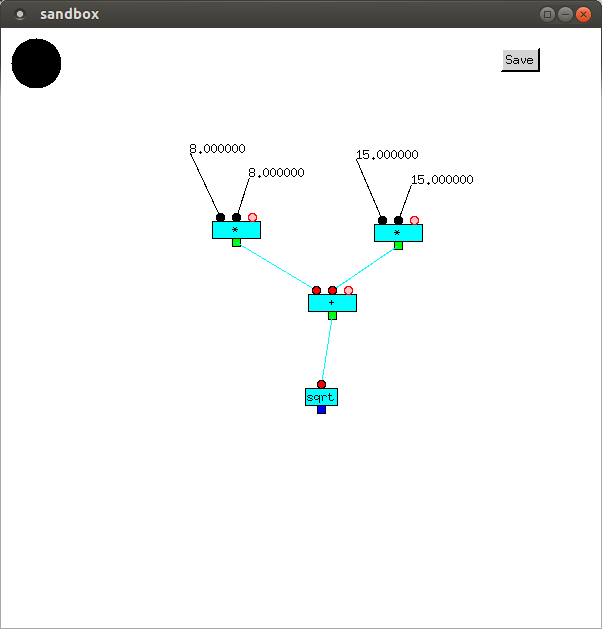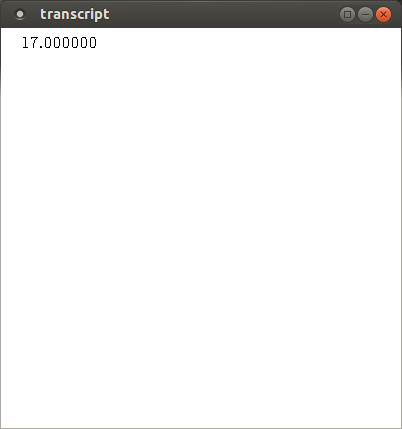# Dataflow

Full Metal Jacket is very different from most other programming languages, as it is graphical, instead of textual, and uses data flow, instead of control flow.

Programs in Full Metal Jacket are inherently parallel. They consist of boxes (or vertices) connected by lines (or edges). Vertices have inputs and (usually) outputs. Outputs are usually connected by edges to inputs on other vertices. During computations, data values flow along these edges. When all the inputs of a vertex have data values, those values are normally removed from the inputs and supplied as arguments to the vertex's function. The values returned by the function are then transmitted from its outputs along the edges connected to them. Execution of vertices doesn't happen in any particular order: if all of the inputs of a vertex are available, it can be executed.

The following program outputs the value of

```        sqrt(8.0 * 8.0 + 15.0 * 15.0)
```

Its output is shown, below it.8.0 and 8.0 are input to one `*`, and 15.0 and 15.0 are input to another `*`. These multiply their inputs together and output 64.0 and 225.0 respectively, which are transmitted to `+`, which adds its inputs, and outputs 289.0, which is transmitted to `sqrt`, which outputs its square root, 17.0.

### Notation

This is a good point to explain the appearance of the code. Vertices, which perform a calculation, are cyan. Inputs are normally red, but if the input is a constant it is shown as black. If a function has a variable number of inputs, new ones are added by clicking on the pink input. Outputs are normally green, but if a vertex's output has been changed to blue, it is output to the transcript. The types of edges are given distinct colors. Here, edges transmitting `Real` values are colored cyan.